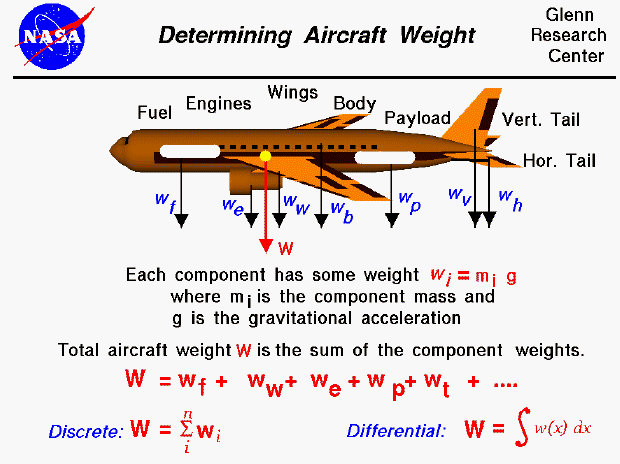Weight is the force generated by the gravitational attraction of the earth on the airplane. The mass (and weight) is actually distributed throughout the airplane and for some problems it is important to know the distribution. But for total aircraft maneuvering, we only need to be concerned with the total weight and the location of the center of gravity. The center of gravity is the average location of the mass of any object.

How do engineers determine the weight of an airplane which they are designing?

In general, determining the weight is a complicated procedure requiring the use of calculus. We'll discuss the general answer at the bottom of this page. This figure shows a simplified version of this calculation which can be used by secondary students. For this figure, we assume that we already know the weight of each of the major components of the airplane (the fuselage, vertical and horizontal tails, wings, engines, fuel, payload, etc.). The individual component weight is simply the mass of the component times the gravitational constant, g , which is 32.2 ft/square sec in English units and 9.8 meters/square sec in metric units. The total weight of the aircraft is then simply the sum of all the individual weights of the components.

We can generalize the addition of component weights. If we had a total of "n" discrete components, the total weight of the aircraft would be the sum of the individual (i) component weights with the index i going from 1 to n. The greek mathematical symbol sigma is used by mathematicians to denote this addition. (Sigma is a zig-zag symbol with the index designation being placed below the bottom bar, the total number of additions placed over the top bar, and the variable to be summed placed to the right of the sigma with each component designated by the index.) So this equation says that the weight of the airplane is equal to the sum of the weight of "n" discrete parts.

But what if the parts are not discrete? What if we had a continuous change of mass from front to back? The continuous change can be computed using integral calculus. The sigma designation is changed to the integral "S" shaped symbol to denote a continuous variation. The discrete weight is replaced with w(x) which indicates that the weight is some function of distance x. If we are given the form of the function, there are methods to solve the integration. If we don't know the actual functional form, we can still numerically integrate the equation using a spread sheet by dividing the distance up into a number of small distance segments and determining the average value of the mass (or weight) over that small segment, then summing up the value.Guided Tours